User’s Guide : Basic Data Analysis : Series : Correlogram

Correlogram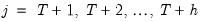This view displays the autocorrelation and partial autocorrelation functions up to the specified order of lags. These functions characterize the pattern of temporal dependence in the series and typically make sense only for time series data. When you select View/Correlogram… the Correlogram Specification dialog box appears.
You may choose to plot the correlogram of the raw series (level) x, the first difference d(x)=x–x(–1), or the second difference
d(x)-d(x(-1)) = x-2x(-1)+x(-2)
of the series.
You should also specify the highest order of lag to display the correlogram; type in a positive integer in the field box. The series view displays the correlogram and associated statistics: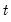Autocorrelations (AC)
The autocorrelation of a series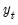at lag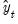is estimated by: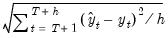(11.19)
where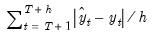is the sample mean of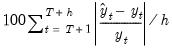. This is the correlation coefficient for values of the series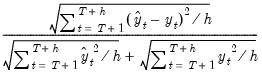periods apart. If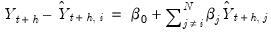is nonzero, it means that the series is first order serially correlated. If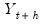dies off more or less geometrically with increasing lag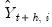, it is a sign that the series obeys a low-order autoregressive (AR) process. If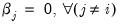drops to zero after a small number of lags, it is a sign that the series obeys a low-order moving-average (MA) process. See “Background” for a more complete description of AR and MA processes.
Note that the autocorrelations estimated by EViews, which follow those in the literature, differ slightly from the theoretical definition of the autocorrelation: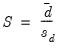(11.20)
where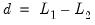. The difference arises since, for computational simplicity, EViews employs the same overall sample mean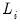as the mean of both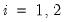and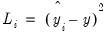. While both formulations are consistent estimators, the EViews formulation biases the result toward zero in finite samples.
The dotted lines in the plots of the autocorrelations are the approximate two standard error bounds computed as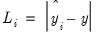. If the autocorrelation is within these bounds, it is not significantly different from zero at (approximately) the 5% significance level.
Partial Autocorrelations (PAC)
The partial autocorrelation at lag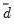is the regression coefficient on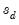when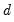is regressed on a constant,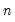. This is a partial correlation since it measures the correlation of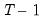values that are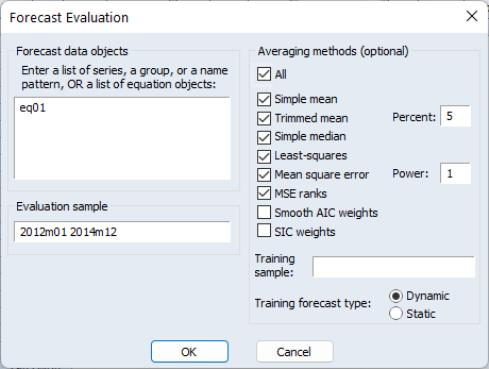periods apart after removing the correlation from the intervening lags. If the pattern of autocorrelation is one that can be captured by an autoregression of order less than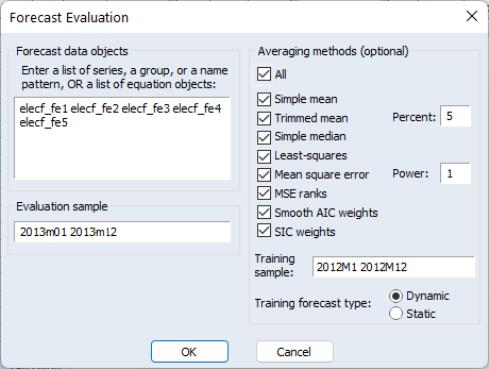, then the partial autocorrelation at lagwill be close to zero.
The PAC of a pure autoregressive process of order, AR(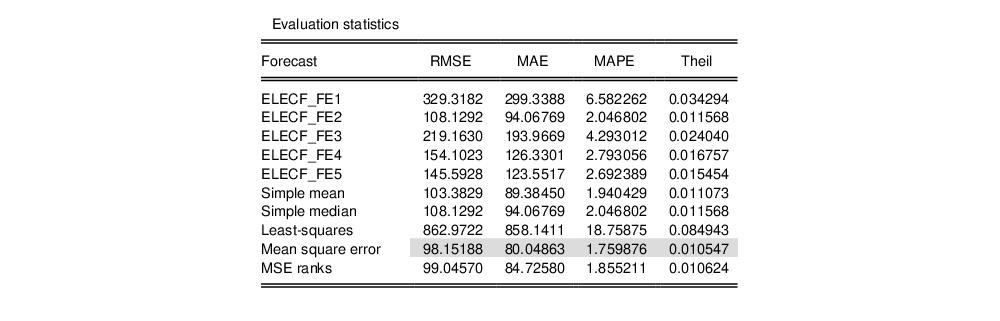), cuts off at lag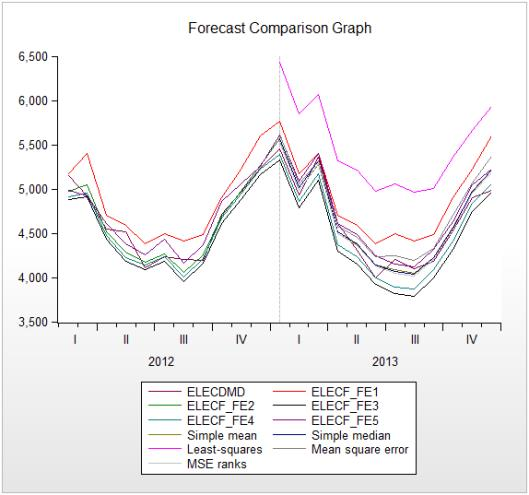, while the PAC of a pure moving average (MA) process asymptotes gradually to zero.
EViews estimates the partial autocorrelation at lag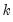recursively by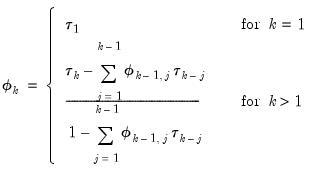(11.21)
where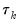is the estimated autocorrelation at lagand where,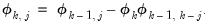(11.22)
This is a consistent approximation of the partial autocorrelation. The algorithm is described in Box and Jenkins (1976, Part V, Description of computer programs). To obtain a more precise estimate of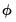, simply run the regression: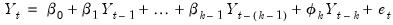(11.23)
where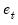is a residual. The dotted lines in the plots of the partial autocorrelations are the approximate two standard error bounds computed as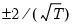. If the partial autocorrelation is within these bounds, it is not significantly different from zero at (approximately) the 5% significance level.
Q-Statistics
The last two columns reported in the correlogram are the Ljung-Box Q-statistics and their p-values. The Q-statistic at lagis a test statistic for the null hypothesis that there is no autocorrelation up to orderand is computed as: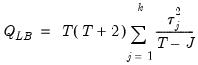(11.24)
where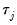is the j-th autocorrelation and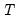is the number of observations. If the series is not based upon the results of ARIMA estimation, then under the null hypothesis, Q is asymptotically distributed as a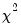with degrees of freedom equal to the number of autocorrelations. If the series represents the residuals from ARIMA estimation, the appropriate degrees of freedom should be adjusted to represent the number of autocorrelations less the number of AR and MA terms previously estimated. Note also that some care should be taken in interpreting the results of a Ljung-Box test applied to the residuals from an ARMAX specification (see Dezhbaksh, 1990, for simulation evidence on the finite sample performance of the test in this setting).
The Q-statistic is often used as a test of whether the series is white noise. There remains the practical problem of choosing the order of lag to use for the test. If you choose too small a lag, the test may not detect serial correlation at high-order lags. However, if you choose too large a lag, the test may have low power since the significant correlation at one lag may be diluted by insignificant correlations at other lags. For further discussion, see Ljung and Box (1979) or Harvey (1990, 1993).